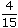# Aptitude - Ratio and Proportion

Exercise : Ratio and Proportion - General Questions
1.
A and B together have Rs. 1210. Ifof A's amount is equal toof B's amount, how much amount does B have?
Rs. 460
Rs. 484
Rs. 550
Rs. 664
Explanation:

 4 A = 2 B 15 5A =2 x 15B 5 4A = 3 B 2A = 3 B 2A : B = 3 : 2.B's share = Rs.1210 x 2= Rs. 484. 5

2.
Two numbers are respectively 20% and 50% more than a third number. The ratio of the two numbers is:
2 : 5
3 : 5
4 : 5
6 : 7
Explanation:

Let the third number be x.

 Then, first number = 120% of x = 120x = 6x 100 5

 Second number = 150% of x = 150x = 3x 100 2Ratio of first two numbers =6x : 3x= 12x : 15x = 4 : 5. 5 2

3.
A sum of money is to be distributed among A, B, C, D in the proportion of 5 : 2 : 4 : 3. If C gets Rs. 1000 more than D, what is B's share?
Rs. 500
Rs. 1500
Rs. 2000
None of these
Explanation:

Let the shares of A, B, C and D be Rs. 5x, Rs. 2x, Rs. 4x and Rs. 3x respectively.

Then, 4x - 3x = 1000x = 1000.B's share = Rs. 2x = Rs. (2 x 1000) = Rs. 2000.

4.
Seats for Mathematics, Physics and Biology in a school are in the ratio 5 : 7 : 8. There is a proposal to increase these seats by 40%, 50% and 75% respectively. What will be the ratio of increased seats?
2 : 3 : 4
6 : 7 : 8
6 : 8 : 9
None of these
Explanation:

Originally, let the number of seats for Mathematics, Physics and Biology be 5x, 7x and 8x respectively.

Number of increased seats are (140% of 5x), (150% of 7x) and (175% of 8x).140 x 5x,150 x 7xand175 x 8x100 100 1007x, 21x and 14x. 2The required ratio = 7x : 21x : 14x 214x : 21x : 28x2 : 3 : 4.

5.
In a mixture 60 litres, the ratio of milk and water 2 : 1. If this ratio is to be 1 : 2, then the quantity of water to be further added is:
20 litres
30 litres
40 litres
60 litres
Explanation:

 Quantity of milk =60 x 2litres = 40 litres. 3

Quantity of water in it = (60- 40) litres = 20 litres.

New ratio = 1 : 2

Let quantity of water to be added further be x litres.

 Then, milk : water =40. 20 + x

 Now,40= 1 20 + x 220 + x = 80x = 60.Quantity of water to be added = 60 litres.